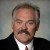# While calculating Pp and Ppk, how do I determine the value of Short term and long term Standard deviation?

ByDr. Mikel Harry

One must necessarily understand that the short-term standard deviation reports on the “instantaneous reproducibility” of a process whereas the long-term standard deviation reflects the “sustainable reproducibility.”  To this end, the short-term standard deviation is comprised of the “within group” sums-of-squares (SSW).  The long-term standard deviation incorporates the “total” sums-of-squares (SST).  Of course, the difference between the two constitutes the “between group” sums-of-squares (SSB).

By employing a rational sampling strategy it is possible to effectively block the noises due to assignable causes from those due to random causes.  In this context, we recognize that SST = SSW + SSB.  By considering the degrees-of-freedom associated with SST and SSW, we are able to compute the corresponding variances and then establish the respective standard deviations.  In the case of a process characterization study, we note that the short-term standard deviation is given by the quantity Sqrt(SSW / g(n – 1)).  The long-term standard deviation is defined as Sqrt(SST / ng– 1).

When computing Cp and Cpk, it is necessary to employ the short-term standard deviation.  This ensures that the given index of capability reports on the instantaneous reproducibility of the process under investigation.  So as to reflect the sustainable reproducibility of the process, the long-term standard deviation must be employed to compute Pp and Ppk.  Oddly enough, many practitioners confuse these two overlapping sets of performance indices.

Handpicked Content:   How do you see the intersection of Six Sigma and Lean?

For more information on this topic, reference Harry, M. J. “The Vision of Six Sigma: A Roadmap for Breakthrough” located at http://www.tristarvisual.com/sixsigma2/index.mgi2.  Also see Harry, M.J. and Lawson, R.J. (1988). Six Sigma Producibility Analysis and Process Characterization. Publication Number 6s-3-03/88. Motorola University Press, Motorola Inc., Schaumburg Illinois.

Handpicked Content:   When should data be excluded from cpk analysis?

As an additional post to your question, let us consider how the shift factor figures into the larger scheme of things.  Generally speaking, the shift factor is added to an estimate of long-term capability in order to remove long-term influences, therein providing an approximation of the short-term capability.  Conversely, the shift factor is subtracted from an estimate of the short-term capability in order to inject long-term influences, thereby providing an approximation of the long-term capability.

For example, if the long-term capability of a process was known to be 4.5s, and we seek to approximate the short-term capability, then 1.5s would be added to 4.5s, therein providing the short-term estimate of 6.0s.  Conversely, if the short-term capability was known to be 6.0s, and we seek to approximate the long-term capability, then 1.5s must be subtracted from 6.0s, therein providing the long-term estimate of 4.5s.